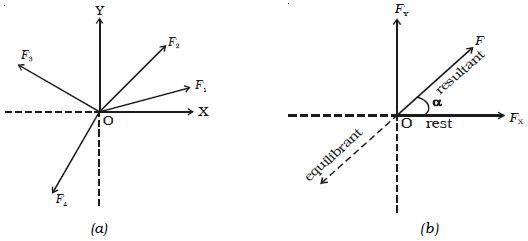Physics

# Explain Equilibrant in terms of Newton’s Second Law of Motion

According to Newton’s second law of motion, a body moves with velocity if it is acted upon by a force. When the body is subjected to number of concurrent forces, it moves in a direction of the resultant force. However, if another force, which is equal in magnitude of the resultant but opposite in direction, is applied to a body, the body comes to rest. Hence. equilibrant of a system of forces is a single force, which acts along with the other forces to keep the body in equilibrium.

Let us consider the forces F1, F2, F3 and F4 acting on a body O as shown in Fig. a. If F is the resultant of all the forces and in order to keep the body at rest, an equal force (known as equilibrant) should act on it in the opposite direction as shown in Fig. b.Fig: Resultant and equilibrant

From Figure b, it is found that, resultant = – equilibrant